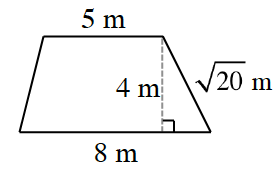### Home > INT2 > Chapter 2 > Lesson 2.1.1 > Problem2-9

2-9.

Determine the area and perimeter of the trapezoid at right.

Recall the formula to find the area of a trapezoid. Try separating the trapezoid
into various shapes when finding missing side lengths.

$A\ =\ \frac{1}{2}\left(b_1\ +\ b_2\right)h$

$A\ =\ 26\ \text{ sq.m},\ P\ =\ 13\ +\ \sqrt{20}+\sqrt{17}\ \approx 21.6\ \text{ m}$